### IMO Shortlist 1999 problem G6

Kvaliteta:
Avg: 0,0
Težina:
Avg: 8,0
Two circles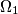$\Omega_{1}$ and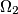$\Omega_{2}$ touch internally the circle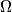$\Omega$ in M and N and the center of$\Omega_{2}$ is on$\Omega_{1}$. The common chord of the circles$\Omega_{1}$ and$\Omega_{2}$ intersects$\Omega$ in$A$ and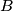$B$.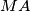$MA$ and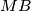$MB$ intersects$\Omega_{1}$ in$C$ and$D$. Prove that$\Omega_{2}$ is tangent to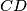$CD$.
Izvor: Međunarodna matematička olimpijada, shortlist 1999